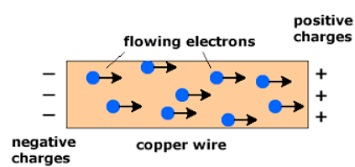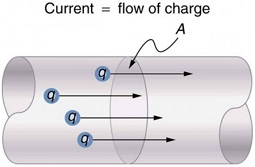# Electric current

## Chemical effect of electric current of Class 8

The electric current is a flow of electric charges (called electrons) in a conductor (metal wire). It is the amount of electric charge passing through a given point of conductor in one second. If a charge of Q coulombs flows through a conductor in time t sec, then the magnitude of the electric current I flows through it is given by:

Q = l/t

The S.I. unit of charge is coulomb (C)

1 C = Charge of 6.25 × 1018 electrons.

### UNIT OF ELECTRIC CURRENT:

The S.I. unit of current is Ampere. When 1 coulomb of charge flows through any cross-section  of a conductor in 1 second, the electric current flowing through it is said to be 1 ampere.

I = V/R

Instrument used to measure current is called ammeter. It should have a very low resistance. It is connected in series in the circuit.

### DIRECTION OF ELECTRIC CURRENT:

We know that there are two types of charges positive charges and negative charges, but electron were not discovered at that time. So, electric current was considered to be the flow of positive charges and the direction of flow of the positive charges were taken to be the direction of electric current. The direction of electric current is from positive terminal of cell to the negative terminal through the circuit.

### FLOW OF ELECTRIC CURRENT IN A WIRE:

An electric current is the flow of electrons in a metal wire (or conductor) when a cell or battery is applied across its ends. A metal wire has plenty of free electrons in it. When the metal wire has not been connected to a source of electricity like a cell or a battery, then the electrons present in it move randomly in all the directions between the atoms of the metal wire as shown in figure.When a source of electricity like a cell or a battery is connected between the ends of the metal wire, then an electric force acts on the electrons present in the wire. Since the electrons are negatively charged, they start moving from negative end to the positive end of the wire. These electrons constitutes the electric current in the wire.